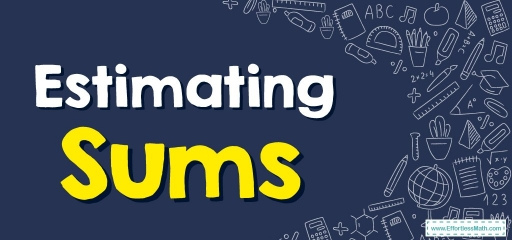# Estimating Sums

In this post, you will learn how to estimate the sum of two or more numbers in a few simple and easy steps.At times whenever we add two large numbers, you do not wish to find out the precise sum. You only wish to get a fast estimate.

A sum can be estimated fast via rounding each addend and then adding these together. Now we’ll demonstrate a few quite easy steps to see the way to estimate a sum.

## Way to estimate sums to the closest tenth

To estimate s sum to the tenth you must follow these three steps:

STEP one: Round the summands to the closest tenth. Don’t forget:

If a numeral ends in $$0, 1, 2, 3$$ or $$4$$, it gets rounded down ?

If a numeral ends in $$5, 6, 7, 8$$ or $$9$$, it gets rounded up  ?

Tip: Unless it is stated differently, you constantly round numbers to the maximum feasible place value.

STEP two: Add the rounded numerals

STEP three: Looking at the overall quantity of rounding, $$3$$ things could occur:

• If  it’s rounded one quantity up as well as one down, the resulting sum is a proper estimation.
• If both the summands were rounded up and then the result of this rounding is more than $$5$$, you take $$10$$ from this estimate.
• If both the summands were rounded down and the quantity of the rounding is more than $$5$$, you add $$10$$ to this estimate.

### Estimating Sums – Example 1:

Estimate sum by rounding each added to the nearest ten.

$$43+58=$$___

Solution:

First, round $$43$$ to the closest ten. Check out the numeral on the right of the tens position. It is $$3$$ and this number is lower than $$5$$. So, $$43$$ is rounded down to $$40$$. Do the same for $$58$$. Then $$58$$ is rounded up to $$60$$. Now add two result together: $$40+60=100$$

### Estimating Sums – Example 2:

Estimate sum by rounding each added to the nearest ten.

$$75+32=$$___

Solution:

First, round $$75$$ to the closest ten. Check out the numeral on the right of the tens position. It is $$5$$. So, $$75$$ is rounded up to $$80$$. Do the same for $$32$$. Then $$32$$ is rounded down to $$30$$. Now add two result together: $$80+30=110$$

## Exercises for Estimating Sums

Estimate sum by rounding each added to the nearest ten.

1. $$\color{blue}{14+35}$$
2. $$\color{blue}{ 46+77 }$$
3. $$\color{blue}{83+38 }$$
4. $$\color{blue}{ 71+95 }$$
1. $$\color{blue}{50}$$
2. $$\color{blue}{ 130 }$$
3. $$\color{blue}{120 }$$
4. $$\color{blue}{ 170 }$$

### What people say about "Estimating Sums - Effortless Math: We Help Students Learn to LOVE Mathematics"?

No one replied yet.

X
30% OFF

Limited time only!

Save Over 30%

SAVE $5 It was$16.99 now it is \$11.99